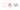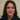# Angle value in CSS explained: degrees, gradians, radians, or turns units

Generally as per MDN the `<angle>` CSS data type represents an angle value expressed in degrees, gradians, radians, or turns.  We can use them in transforms and gradients.

## Degrees, gradians, radians, or turns

Practically we could use different units  for an angle rather than just using degrees. Basically this is how they compare to each other:

``````A full angle:
360deg = 400grad = 1turn ≈ 6.2831853rad``````

Therefor we can calculate them based on this and use them accordingly.

## Turn unit

Besides being just another unit the turn unit is just the perfect choice once we need to specify the rotation unit:

``````.example {
transform: rotate(1turn);
}``````

So the one turn means we want the item to have a one full turn. It is the most intuitive choice in this case.

## Radians unit

A full circle contains 2p radians.  Generally “pi” is equal to 3.14159265. So that’s exactly half a circle in terms of radians. So we double that and we get 6.2831853rad to get a full circle.The unit identifier for radians is `rad`.

``````.example {
transform: rotate(6.2831853rad);
}``````

## Gradians unit

As an explanation gradians also known as “gons” or “grades” are abbreviated using the string “grad” appended to the unit value. A full circle has 400 gradians, which would be the equivalent to 360 degrees.

``````.example {
transform: rotate(400grad);
}``````

Like turn we could use gradians with round numbers to calculate the turns specially.

## Degrees unit

Finally our old good friend degrees unit. We have been use them everywhere and in different use cases. But maybe it is time to make the right choice based on the need in the code. If it is a rotation the turn makes a perfect choice. In gradient backgrounds gradian might be the best or to make easy calculations we could also use them. In some cases we might find a usage for radians too. But it is good to be aware of these data value units.

Thank you for reading.

© 2020This site is created and maintined by Azadeh Faramarzi , A passionate developer, sport and code lover who loves to share and write when she has some time.